Courses

# RD Sharma Solutions -Ex-6.6, Fractions, Class 6, Maths Class 6 Notes | EduRev

## RD Sharma Solutions for Class 6 Mathematics

Created by: Abhishek Kapoor

## Class 6 : RD Sharma Solutions -Ex-6.6, Fractions, Class 6, Maths Class 6 Notes | EduRev

The document RD Sharma Solutions -Ex-6.6, Fractions, Class 6, Maths Class 6 Notes | EduRev is a part of the Class 6 Course RD Sharma Solutions for Class 6 Mathematics.
All you need of Class 6 at this link: Class 6

Q1. Reduce each of the following fractions to its lowest term (simplest form):
i)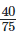ii)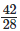iii)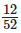iv)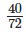v)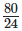vi)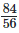Ans : i)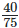Factors of 40 are 1 , 2 , 4 , 5 , 8 , 10 , 20 and 40.
Factors of 75 are 1 , 3 , 5 , 15 and 75.
Common factors of 40 and 75 are 1 and 5 . HCF = 5
Divide both the numerator & denominator by 5. 40÷575÷5 =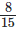Therefore, the simplest form obtained is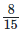(ii)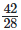Factors of 42 are 1 , 2 , 3 , 6 , 7 , 14 , 21 , 42
Factors of 28 are 1 , 2 , 4 , 7 , 14 , 28
Common factors of 42 & 28 are 1 , 2 and 4. HCF = 4
Divide both the numerator & denominator by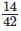÷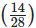÷14=32
Therefore, the simplest form obtained is 32
(iii)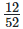Factors of 12 are 1 , 2 , 3 , 4 , 6 and 12. Factors of 52 are 1 , 2 , 4 , 13 , 26 and 52.
Common factors of 12 and 52 are 1 , 2 and 4 HCF = 4
Divide both the numerator & denominator by 4.
On solving the above we have ,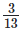Therefore, the simplest form obtained is(iv)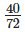Factors of 40 are 1 , 2 , 4 , 5 , 8 , 10 , 20 , 40
Factors of 72 are 1 , 2 , 3 , 4 , 6 , 8 , 9 , 12 , 18 , 24 , 36 , 72
Common factors of 40 and 72 are 1 , 2 , 4 and 8. HCF = 8
Divide both the numerator & denominator by 8.
On solving the above equation, we have :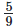Therefore, the simplest form obtained is(v)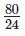Factors of 80 are 1, 2, 4, 5, 8, 10, 16, 20, 40 and 80. Factors of 24 are 1, 2, 3, 4, 6, 8, 12 and 24.
Common factors of 80 and 24 are 1 , 2 , 4 , 8 HCF = 8
Divide both the numerator & denominator by 4.
On solving the above equation, we have :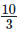Therefore, the simplest form obtained isvi)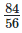Factors of 84 are 1, 2, 3, 4, 6, 7, 12, 14, 21, 28, 42 and 84. Factors of 56 are 1, 2, 4, 7, 8, 14, 28 and Common factors of 84 & 56 are 1, 2, 4, 7, 14 and 28. HCF = 28 Divide both the numerator & denominator by 28
On solving the above equation, we have :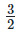Therefore, the simplest form obtained isQ2. Simplify each of the following to its lowest form :
i)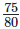ii)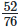iii)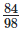iv)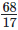v)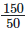vi)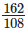Ans : i)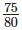Factors of 75 are 1 , 3 , 5 , 15 , 25 and 75. Factors of 80 are 1, 2 , 4 , 5 , 8 , 10 , 12 , 16 , 20 , 40 and 80.
Common factors of 75 and 80 are 1 and 5. HCF of 75 and 80 is 5.
Dividing both the numerator and denominator by 5, we get: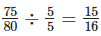Therefore, the simplest form obtained is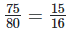ii)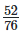Factors of 52 are 1 , 2 , 4 , 13 , 26 and 52. Factors of 76 are 1, 2 , 4 , 19 , 38 and 76.
Common factors of 52 and 76 are 1 , 2 and 4. HCF of 52 and 76 is 4.
Dividing both the numerator and denominator by 5, we get :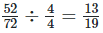Therefore, the simplest form obtained is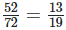iii)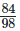Factors of 84 are 1 , 2 , 3 , 4 , 6 , 7 , 12 , 14 , 21 , 28 , 42 and 84. Factors of 98 are 1, 2 , 7 , 14 , 49 and 98.
Common factors of 84 and 98 are 1 , 2 , 7 and 14. HCF of 84 and 98 is 14
Dividing both the numerator and denominator by 5, we get :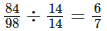Therefore, the simplest form obtained is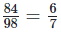iv)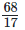Factors of 68 are 1 , 2 , 4 , 17 , 34 and 68. Factors of 17 are 1 and 17.
Common factor of 68 and 17 is 17 HCF of 68 and 17 is 17
Dividing both the numerator and denominator by 5, we get :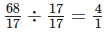Therefore, the simplest form obtained is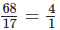v)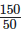Factors of 150 are 1 , 2 , 3 , 5 , 6 , 10 , 15 , 25 , 50 and 150. Factors of 50 are 1 , 2 , 5 , 10 , 25 and 50 .
Common factor of 150 and 50 is 50 HCF of 150 and 50 is 50
Dividing both the numerator and denominator by 50, we get :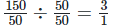Therefore, the simplest form obtained is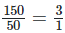vi)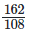Factors of 162 are 1 , 2 , 3 , 6 , 9 , 18 , 27 , 54 , 81 and 162 .
Factors of 108 are 108, 1 , 2 , 3 , 4 , 6 , 9 , 12 , 18 , 27 and 54 .
Common factor of 162 and 108 are 1 , 2 , 3 , 6 , 9 , 18 , 27 , 54 HCF of 162 and 108 is 54
Dividing both the numerator and denominator by 54, we get :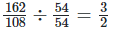Therefore, the simplest form obtained is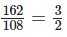103 docs

,

,

,

,

,

,

,

,

,

,

,

,

,

,

,

,

,

,

,

,

,

,

,

,

,

,

,

,

,

,

;